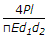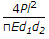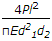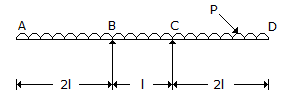# Civil Engineering - UPSC Civil Service Exam Questions

21.

Which one of the following would contain water with the maximum amount of turbidity ?

 A. Lakes B. Oceans C. Rivers D. Wells

Explanation:

No answer description available for this question. Let us discuss.

22.

The following steps are necessary to obtain sufficient accuracy with the tape :
1. Keeping uniform tension on tape for each measurement:
2. "Breaking" tape on slopes are necessary to keep the tape level.
3. Keeping accurate count of the stations.
4. Keeping the tape on the line being measured.
The correct sequence of these steps is

 A. 4, 2, 1, 3 B. 2, 3, 4, 1 C. 4, 1, 2, 3 D. 3, 2, 1, 4

Explanation:

No answer description available for this question. Let us discuss.

23.

A bar of length l is uniformly tapering from a diameter d1 at one and to a diameter d2 at the other end. The bar is subjected to an axial tensile loads. What is the total elongation (where E = Young's modulus of elasticity. )

 A.B.C.D. 4 +Explanation:

No answer description available for this question. Let us discuss.

24.

A welded steel plate girder consisting of two flange plates of 350 mm x 16 mm and a web plate of 1000 mm x 6 mm, requires

 A. nostiffeners B. vertical stiffeners C. intermediate vertical stiffeners D. vertical and horizontal stiffeners

Explanation:

No answer description available for this question. Let us discuss.

25.

ABCD is a beam of length 5l which is supported at B and C (having supported length BC = 'l') and having two equal overhangs AB and CD of length 2l each. It carries a u.d.l. of intensity p per unit length throughout the beam as shown in the given figure.
The points of contraflexure will occurA. at B and C B. no where in the beam C. at the mid-point of BC D. at the mid-points of AB and CD